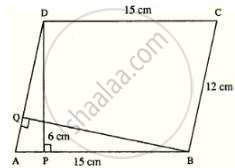# A Parallelogram Has Sides of 15 Cm and 12 Cm; If the Distance Between the 15 Cm Sides is 6 Cm; Find the Distance Between 12 Cm Sides. - Mathematics

Sum

A parallelogram has sides of 15 cm and 12 cm; if the distance between the 15 cm sides is 6 cm; find the distance between 12 cm sides.

#### SolutionBase, AB = 15 cm

Distance between 15 cm sides

i.e. height DP = 6 cm

∴ Area of ||gm = Base × height

= AB × DP

= 15 × 6 = 90 cm2

Let BQ be the distance between 12 cm sides

∴ AD × BQ = area of ||gm ABCD

∴ 12 × BQ = 90

BQ = 90/12

BQ = 15/2 = 7.5 cm

Concept: Area of a Parallelogram
Is there an error in this question or solution?

#### APPEARS IN

Selina Concise Mathematics Class 8 ICSE
Chapter 20 Area of a Trapezium and a Polygon
Exercise 20 (C) | Q 4 | Page 232